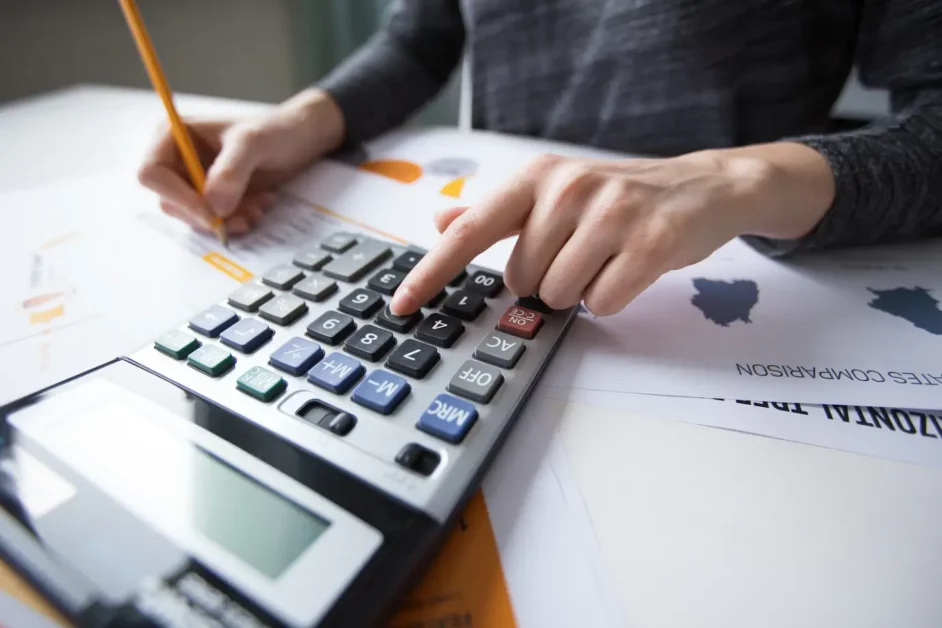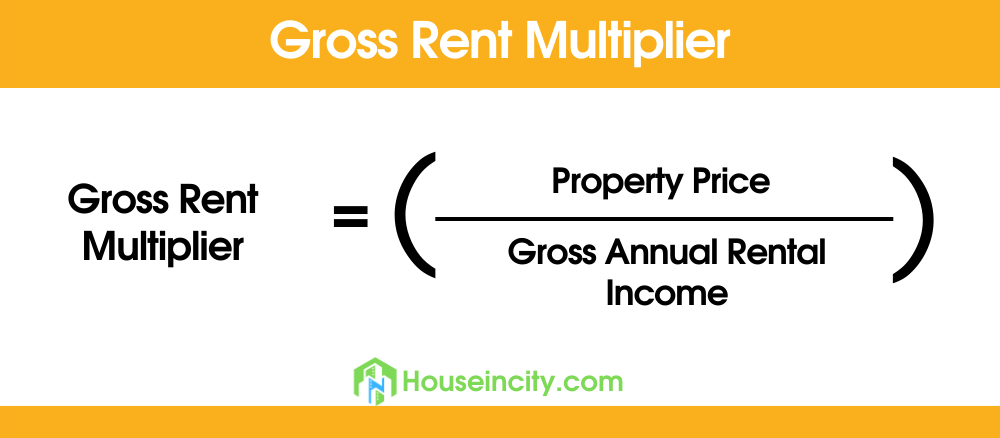# The Gross Rent Multiplier: What It Is and How to Use It

The gross rent multiplier (GRM real estate) is a calculation used to determine the value of a property. It takes into account the amount of rent that can be generated by the property and compares it to the purchase price of the property. This number can be used to help investors decide whether or not a particular property is worth purchasing.

In this article, we will discuss what GRM is and how to use it!

Contents

## What is Gross Rent Multiplier?

The gross rent multiplier is a measure of the relationship between the sale price of a property and the annual gross rents that it could command.

## How to Calculate GRM?To calculate the gross rent multiplier, you will need to divide the sale price of the property by the annual gross rent. So, using the gross rent multiplier formula, if a property is selling for \$100,000 and it could generate \$20,000 in annual rent, then the GRM would be 100,000/20,000, or five.

## What is a Good Gross Rent Multiplier?

There is no definitive answer to this question as it will vary depending on the market you are in and what your investment goals are. However, as a general rule of thumb, a lower gross rent multiplier is better than a higher one. For instance, a GRM of four would be considered better than a GRM of six.

## How to Use Gross Rent Multiplier

There are a few different ways that you can use the gross rent multiplier to help you make investment decisions. First, you can use it as a general guide to see if a property is overpriced or underpriced.

If the GRM is much higher than similar properties in the area, then it may be overpriced. On the other hand, if the GRM is lower than similar properties, it may be a good deal.

You can also use the gross rent multiplier to help you estimate what your return on investment (ROI) could be. To do this, you will need to multiply the GRM by the annual gross rent.

For example, if you purchase a property for \$100,000 with a GRM of four and it generates \$20,000 in annual rent, your ROI would be \$20,000*0.04, or 800%.

Finally, you can use the gross rent multiplier to help you determine how much you should offer for a property. If you know what the GRM is for similar properties in the area, you can use that information to haggle with the seller and try to get the best price possible.

## Creating a Gross Rent Multiplier Grading Scale

Now that we know what the gross rent multiplier is and how to calculate it, let’s create a grading scale. This will help us determine whether a particular property is a good deal or not.

A GRM of:

• 0-Two = A good deal
• Three = A fair deal
• Four = Not a bad deal
• Five = Getting expensive
• Six = Expensive
• Seven = Very expensive
• Eight+ = Extremely expensive

Typically, the higher the GRM, the higher the likelihood that a property is newer with modern features and amenities. However, keep in mind that this is just a general guide and you should always do your own research to find out what is considered a good GRM in your specific market.

## The Pros and Cons of Using Gross Rent Multiplier

There are both pros and cons to using the gross rent multiplier. One of the main advantages is that it is a quick and easy way to estimate the value of a property. It doesn’t require a lot of research or analysis, which can be time-consuming.

Additionally, the gross rent multiplier can be useful in markets where comparable sales data is not readily available.

However, there are also some drawbacks to using the gross rent multiplier. One of the main disadvantages is that it doesn’t take into account all of the factors that affect property value.

For example, it does not consider the condition of the property, the location, or the quality of the tenants. Additionally, the gross rent multiplier is based on historical data, so it may not be accurate in a rapidly changing market.

Despite its flaws, the gross rent multiplier is still a valuable tool that can be used to estimate the value of a property. When used correctly, it can help you make investment decisions and get the best price possible.

## Final Verdict

If you are looking to invest in real estate, understanding and using the gross rent multiplier can be a helpful tool in your decision-making process. Do your research and remember to take into account your own investment goals when considering a property.

Happy hunting!